# Kinetic and Potential energy

Introduction

Good day learners

Did you know that where you are sitting now, you have potential energy and if you get up and walk to the kitchen for a glass of water,  you have kinetic energy?

Even the cup in the cupboard above kettle has potential energy. How so you may ask yourself, if it is only an object?

While the answer is in the information below. Don't forget to watch the video as well for a better understanding.

Have fun on the rollercoaster below.

1. WHAT IS POTENTIAL ENERGY?
The energy an object has due it’s position (height) above the Earth’s surface relative to a reference point.

1.1 Potential energy is proportional to the mass of an object.

𝑃𝐸 ∝ 𝑚𝑎𝑠𝑠

Therefor, the greater the mass, the greater the potential energy.

1.2 Potential energy is also proportional to the height of an object.

𝑃𝐸 ∝ h𝑒𝑖𝑔h𝑡

Therefor, the greater the height, the greater the potential energy.

The formula used to calculate potential energy is as follows:

EP = m g h where;

m = mass (kg)

g = (gravitational acceleration 9,8 m.s-2

h = height (meters)​​​​

2. What is kinetic energy?

The energy an object has/stores due to its motion.

Kinetic energy is proportional to the mass of an object.

KE ∝ m

The greater the mass of an object, the greater the greater the kinetic energy.

Kinetic energy is proportional to the velocity of an object.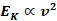The faster the motion, the greater the kinetic energy.

The formula for Kinetic energy: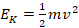(SI-UNITS)

Where;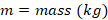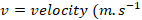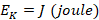Watch the video below to distinguish between potential and kinetic energy.

Watch the video below and follow the links to assist in answering the questions below and refer to the information in the introduction for clarity or formulas.

Read the question carefully and note what is asked and what is given.

Ensure that the correct SI-units are used and that conversions are done accurately.

All answers must be to 2 decimal places. Round off at end of the sum for accuracy.

1. A motorbike is travelling at a speed v, has a kinetic energy E. If the speed of the motorbike increases to 3v the kinetic energy will be…

A. 3E

B. 13 E

C. 6E

D. 9E

2. The SI-unit for gravitational potential energy is…

A. kg.m/s¹

B. kg.m/s²

C. kg.m²s/²

D. kg/m¹.s¹

3. A man carries a briefcase of mass 7 kg while he climbs a ladder from the ground to point P which is 3.1 m above the ground. When he reached point P, the man drops the briefcase.

3.1 Calculate how much gravitational potential energy the briefcase has gained when it reached point P.

3.2 Determine the kinetic energy of the briefcase when it hits the floor. Justify your answer.

3.3 Describe the relationship between an object’s potential energy and

a) it’s mass

b) height above reference point

4. A pot plant with a mass of 3 kg falls off a balcony 17 m from the ground. At 4 m after falling from the balcony, the pot plant has a velocity of 7, 34 m/s¹. Ignore the effect of air resistance.

4.1 Calculate the gravitational potential energy of the pot plant before it begins to fall.

4.2 Calculate the mechanical energy of the pot plant after it has fallen 4 m.

4.3 Calculate the speed with which the pot plant hits the ground.

Process

Watch the video in the Introduction section as brief description to potential and kinetic energy.

Read through the notes provided for additional information on the topic of Energy.

Know the relationship between height, mass and velocity to potential and kinetic energy respectively.

Answer question 3 and question 4 to 2 decimal places.

Evaluation
QUESTION METHOD

SUBSTITUTION

(SI - UNIT)

ACCURACY DEFINTION/RELATIONSHIP TOTAL
Q1 1 1 1   3
Q2 1 1 1   3
Q3 1 2 1 2 6
Q4 1 2 1 2 6

Conclusion

In the lesson learners were able to:

• distinguish between potential and kinetic energy based on an object's position and motion;
• proof the relationship between potential energy, mass and height.
• proof the relationship between kinetic energy, mass and velocity.
• substitute variables into the applicable formula to calculate the energy.

Credits

Watch this video above  in preparation for the next lesson on Law of Conservation on Energy of a system.

Teacher Page

Learners are now aware that objects have potential and kinetic energy based on their position relevant to a reference point and if in their motion.

This information can now used to further explore the law of conservation of mechanical energy using examples such as skateboarder on a ramp or the pendulum.